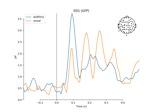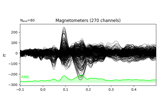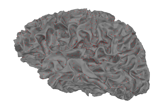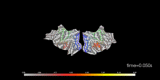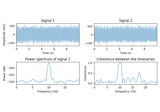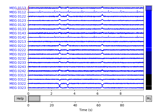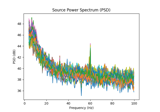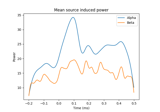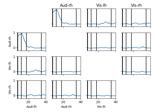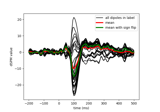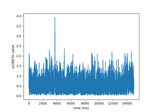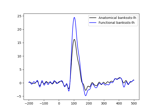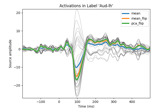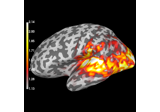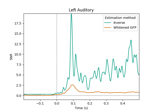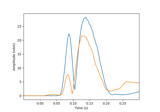# mne.minimum_norm.InverseOperator¶

class mne.minimum_norm.InverseOperator[source]

InverseOperator class to represent info from inverse operator.

Methods

 `__contains__`(key, /) True if the dictionary has the specified key, else False. `__getitem__` x.__getitem__(y) <==> x[y] Implement iter(self). Return len(self). Return a copy of the InverseOperator. `fromkeys`(iterable[, value]) Create a new dictionary with keys from iterable and values set to value. `get`(key[, default]) Return the value for key if key is in the dictionary, else default. `pop`(k[,d]) If key is not found, d is returned if given, otherwise KeyError is raised Remove and return a (key, value) pair as a 2-tuple. `setdefault`(key[, default]) Insert key with a value of default if key is not in the dictionary. `update`([E, ]**F) If E is present and has a .keys() method, then does: for k in E: D[k] = E[k] If E is present and lacks a .keys() method, then does: for k, v in E: D[k] = v In either case, this is followed by: for k in F: D[k] = F[k]
__contains__(key, /)

True if the dictionary has the specified key, else False.

__getitem__()

x.__getitem__(y) <==> x[y]

__iter__(/)

Implement iter(self).

__len__(/)

Return len(self).

clear() None.  Remove all items from D.
copy()[source]

Return a copy of the InverseOperator.

fromkeys(iterable, value=None, /)

Create a new dictionary with keys from iterable and values set to value.

get(key, default=None, /)

Return the value for key if key is in the dictionary, else default.

items() a set-like object providing a view on D’s items
keys() a set-like object providing a view on D’s keys
pop(k[, d]) v, remove specified key and return the corresponding value.

If key is not found, d is returned if given, otherwise KeyError is raised

popitem(/)

Remove and return a (key, value) pair as a 2-tuple.

Pairs are returned in LIFO (last-in, first-out) order. Raises KeyError if the dict is empty.

setdefault(key, default=None, /)

Insert key with a value of default if key is not in the dictionary.

Return the value for key if key is in the dictionary, else default.

update([E, ]**F) None.  Update D from dict/iterable E and F.

If E is present and has a .keys() method, then does: for k in E: D[k] = E[k] If E is present and lacks a .keys() method, then does: for k, v in E: D[k] = v In either case, this is followed by: for k in F: D[k] = F[k]

values() an object providing a view on D’s values

## Examples using `mne.minimum_norm.InverseOperator`¶